Matplotlib

# Matplotlib

matplotlib 提供了一整套和Matlab相似的命令API，十分适合交互式地进行绘图。而且也可以方便的将它最为绘图控件，嵌入GUI应用中。matplotlib可以绘制多种形式的图包括普通的线图，直方图，饼图，散点图以及误差曲线等；可以比较方便的定制图形的各种属性不如图线的颜色，粗细，字体的大小等，它能够很好地支持一部分TeX排版命令，可以美观的显示图中的数学公式

## 1 pylab介绍

matplotlib种包含几十个不同的模块，如matlob，mathtext，finance，dates等，而pylab则是其中最常用的模块

### 1.0 设置图片渲染途径

IPython 支持两种形式的绘图

``%matplotlib inline``

``%matplotlib qt``

### 1.1 中文显示问题解决方案

``````from pylab import mpl
mpl.rcParams['font.sans-serif'] = ['FangSong'] # 指定默认字体
mpl.rcParams['axes.unicode_minus'] = False # 解决保存图像时‘-’显示为方块的问题  ``````

### 1.2绘制最简单的图形

``````import numpy as np
import matplotlib.pyplot as plt
#plt.rcParams['figure.dpi'] = 100 #分辨率
plt.rcParams['figure.dpi'] = 100 #分辨率

x = np.arange(0, 2 * np.pi, 0.01)
y = np.sin(x)
plt.plot(x, y)
plt.show()  ``````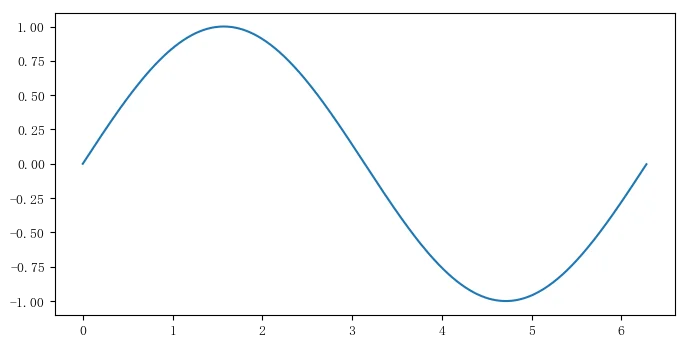### 1.3 设置标题、坐标名称、坐标轴范围

``````import numpy as np
import matplotlib.pyplot as plt
from pylab import mpl
mpl.rcParams['font.sans-serif'] = ['FangSong']
mpl.rcParams['axes.unicode_minus'] = False
x = np.arange(0, 2*np.pi, 0.01)
y = np.sin(x)
plt.plot(x, y)
plt.title(u'正弦曲线', fontdict={'size':20}) # 设置标题
plt.xlabel(u'弧度', fontdict={'size':16})  # 显示横轴名称
plt.ylabel(u'正弦值',fontdict={'size':16}) # 显示纵轴名称
plt.axis([-0.1*np.pi, 2.1*np.pi, -1.1, 1.1]) # 设置坐标轴范围
plt.show()  ``````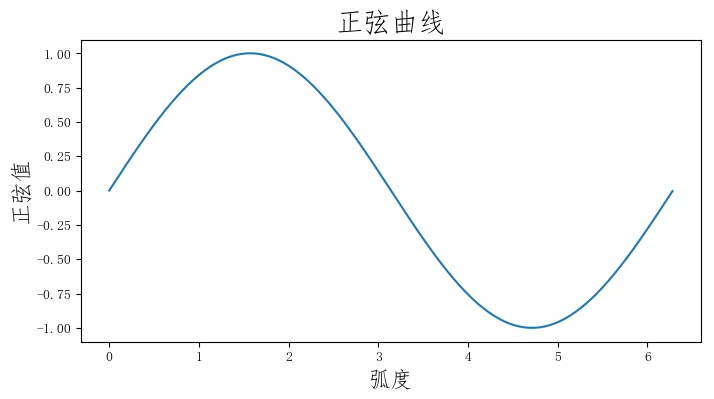### 1.4 设置点和线的样式、宽度、颜色

plt.plot函数的调用形式如下：

``````plot(x, y, color="green", linestyle="dashed", linewidth=1, marker='o', markerfacecolor='blue', markersize=6)
plot(x, y, c='g', ls='---', lw=1, marker='o', mfc='blue', ms=6)  ``````
1. color 指定线的颜色， 可简写为‘c’。颜色的选项为：
• 蓝色 ‘b’
• 绿色 ‘g’
• 红色 ‘r’
• 墨绿 ‘c’
• 洋红 ‘m’
• 黄色 ‘y’
• 黑色 ‘k’
• 白色 ‘w’
• 灰度表示 e.g. 0.75([0, 1]内的任意浮点数)
• RGB表示法 e.g. ‘#2F4F4F’ 或 (0.18, 0.31, 0.31)
2. linestyle 指定线型, 可简写为’ls’
• 实线： ‘-’
• 虚线： ‘–’
• 虚点线: ‘-.’
• 点线：‘:’
• 无： ‘’或‘None’
3. linewidth 指定线的宽度, 可简写为’lw’
4. marker 描述数据点的形状
• 点线 ‘.’
• 点线 ‘o’
• 加号 ‘+’
• 叉号 ‘x’
• 上三角 ‘^’
• 上三角 ‘v’
5. markerfacecolor 指定数据点标记的表面颜色， 可简写为 ‘mfc’
6. markersize 指定数据点标记的大小， 可简写为‘ms’

### 1.5 文本标注和图例

``````import numpy as np
import matplotlib.pyplot as plt
from pylab import mpl
mpl.rcParams['font.sans-serif'] = ['FangSong']
mpl.rcParams['axes.unicode_minus'] = False
x = np.linspace(-4, 4, 200)
f1 = np.power(10, x)
f2 = np.power(np.e, x)
f3 = np.power(2, x)
plt.plot(x, f1, 'r', ls='-', lw=2, label="\$10^x\$")
plt.plot(x, f2, 'b', ls='--', lw=2, label='\$e^x\$')
plt.plot(x, f3, 'g', ls=':', lw=2, label='\$2^x\$')
plt.axis([-4, 4, -0.5, 8])
plt.text(1, 7.5, r'\$10^x\$', fontsize=16)
plt.text(2.2, 7.5, r'\$e^x\$', fontsize=16)
plt.text(3.2, 7.5, r'\$2^x\$', fontsize=16)
plt.title("幂函数曲线", fontsize=16)
plt.legend(loc='upper left')
plt.show()  ``````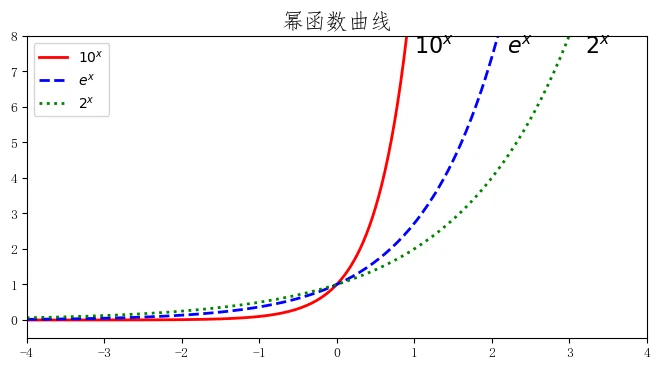## 2 绘制多轴图

``subplot(numRows, numCols, plotNum)  ``

subplot 将整个绘图区域等分为numRows行*numCols列个子区域，然后按照从左到右，从上到下的顺序对每个子区域进行编号，左上的子区域的编号为1。如果numRows，numCols和plotNUm这三个数都小于10的话，可以把他们缩写为一个整数，例如subplot(323)和subplot(3,2,3)是相同的。subplot在plotNum指定的区域中创建一个轴对象。如果新创建的轴和之前创建的轴重叠的话，之前的轴将会被删除。

``````import matplotlib.pyplot as plt
plt.subplot(221) # 两行两列的第一个位置
plt.axis([-1, 2, -1, 2])
plt.axhline(y=0.5, color='b')
plt.axhline(y=0.5, xmin=0.25, xmax=0.75, color='r')

plt.subplot(222) # 两行两列的第二个位置
plt.axis([-1, 2, -1, 2])
plt.axvline(x=0, ymin=0, lw=4, c='r')
plt.axvline(x=1.0, ymin=-0.5, ymax=0.5, lw=4, c='g')

plt.subplot(212) # 两行一列的第二个位置
plt.axis([-1, 2, -1, 2])
plt.axvspan(1.25, 1.55, fc='g', alpha=0.5)
plt.axhspan(0.25, 0.75, facecolor='0.5', alpha=0.5)
plt.show()  ``````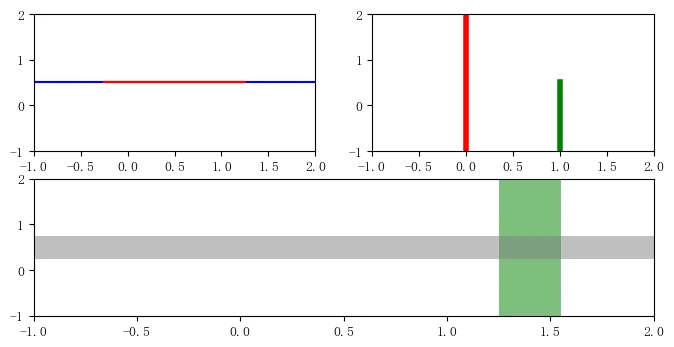## 3 常用；绘图类型

### 3.1 直方图

``````import numpy as np
import matplotlib.pyplot as plt
data = np.random.normal(5.0, 3.0, 1000)
plt.hist(data)
bins = np.arange(-5, 16, 1)
plt.hist(data, bins) # 使用自定义的分段区域
plt.show()  ``````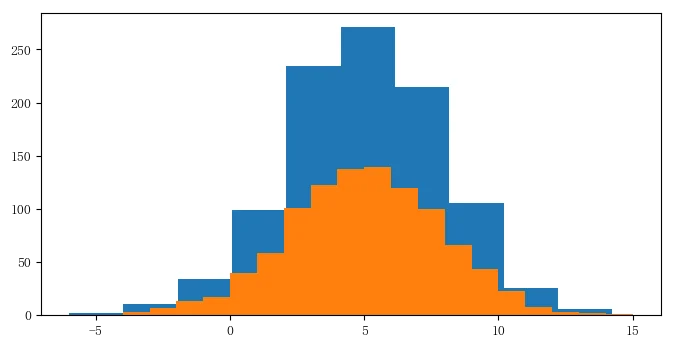### 3.2 散点图

plt.scatter函数的调用形式如下：

``scatter(x, y, s=None, c=None, marker=None, cmap=None, norm=None, vmin=None, vmax=None, alpha=None, linewidths=None, verts=None, edgecolors=None, data=None, **kwargs)  ``
• x ，y两个参数是数组， 分别指定每个点的X轴和Y轴坐标。
• s参数指定点的大小，值和点的面积成正比，它可以是一个数，指定所有点的大小， 也可以是数组分别指定每个点的大小。
• c参数指定每个点的颜色，可以是数值或数组。这里使用一维数组为每个点指定一个数值。通过颜色映射表，每个值都会与一个颜色相对应。默认的颜色映射表中蓝色与最小值相对应，红色与最大值对应。当c参数的形状是(N, 3)或(N, 4)的二维数组时则直接表示每个点的RGB颜色。
• marker参数设置点的形状，可以是表示形状的字符串，也可以是表示多边形的两个元素的元组， 第一个元素表示多边形的边数，第二个参数表示多边形的样式，取值范围为0、1、2、3。0表示多边形，1表示星形，2表示放射形，3表示忽略边数显示为圆形。
• alpha参数设置点的透明度。
• facecolors参数为‘none’时表示散列点没有填充色
``````import numpy as np
import matplotlib.pyplot as plt
x = np.random.rand(50)
y = np.random.rand(50)
area = np.pi * (15 * np.random.rand(50)) ** 2
color = 2 * np.pi * np.random.rand(50)
plt.scatter(x, y, s=area, c=color, alpha=0.5, cmap=plt.cm.hsv)
plt.show()   ``````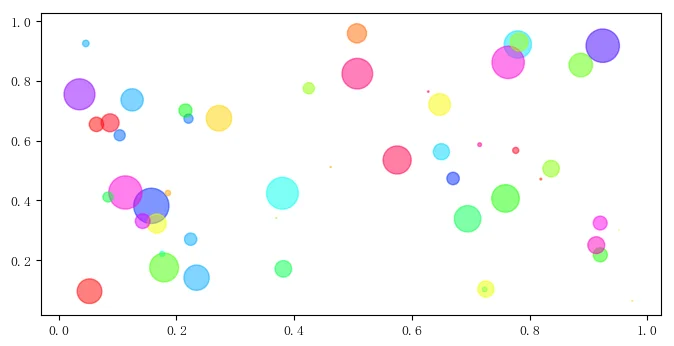### 3.3 梯形图、柱状图、填充图

``````import numpy as np
import matplotlib.pyplot as plt
n = np.array([0, 1, 2, 3, 4, 5])
x = np.linspace(-0.75, 1., 100)
plt.subplot(131)
plt.step(n, n**2, lw=2) # 梯形图
plt.subplot(132)
plt.bar(n, n**2, align='center', width=0.5, alpha=0.5) # 柱状图
plt.subplot(133)
plt.fill_between(x, x**2, x**3, color="green", alpha=0.5) # 填充图
plt.show()  ``````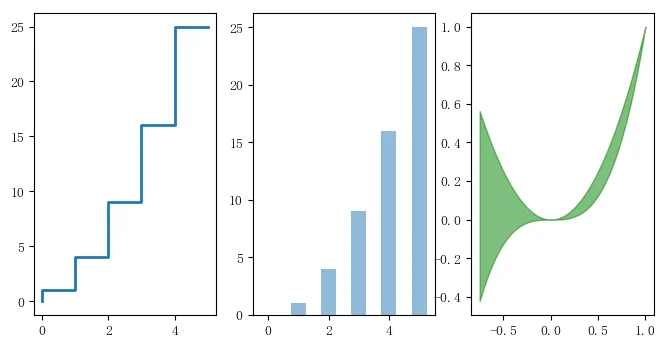### 3.4 对数坐标

plot()所绘制图表的X-Y轴都是算数坐标。绘制对数坐标图的函数有三个：semilogx()、semilogy()和loglog(),他们分别绘制X轴为对数坐标、Y为对数坐标以及两个轴都为对数坐标时的图表。

``````import numpy as np
import matplotlib.pyplot as plt
x = np.linspace(0, 3, 100)
y = np.power(2, np.power(2, x))
plt.subplot(121)
plt.semilogy(x, y, '-r')
plt.subplot(122)
plt.plot(x, y, '--g')
plt.show()  ``````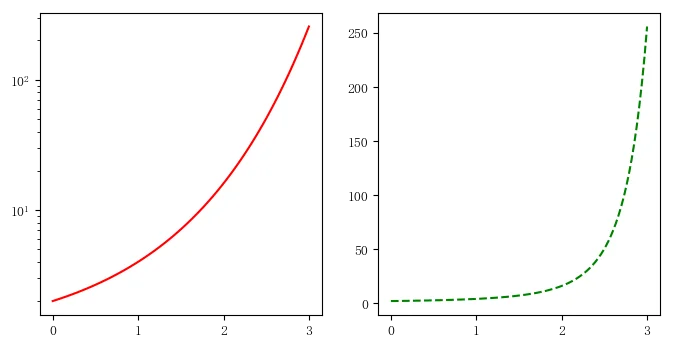### 3.5 极坐标绘图

``````import numpy as np
import matplotlib.pyplot as plt
theta = np.arange(0, 2*np.pi, 0.02)
plt.polar(theta, 1.4*np.cos(5*theta), '--', lw=2)
plt.polar(theta, 1.8*np.cos(4*theta), lw=2, color='g')
plt.show()

plt.subplot(111, polar=True)
plt.plot(theta, 1.4*np.cos(5*theta), ':', lw=2, )
plt.show()  ``````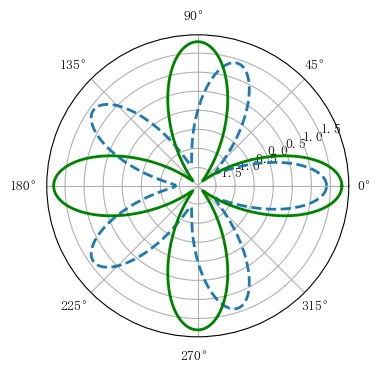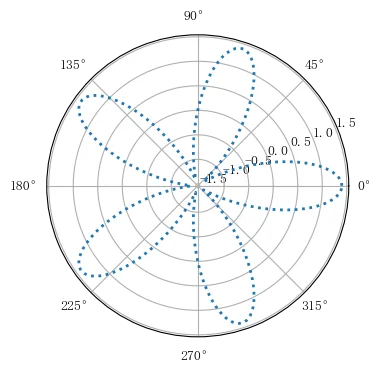## 4 2D绘图

### 4.1 等值线图

``````import numpy as np
import matplotlib.pyplot as plt
y, x = np.ogrid[-2:2:200j, -3:3:300j]
z = x * np.exp(- x**2 - y**2)
extent = [np.min(x), np.max(x), np.min(y), np.max(y)]
plt.subplot(121)
cs = plt.contour(z , 10, extent=extent, cmap=plt.cm.cool)
plt.clabel(cs) # 添加等值线的值
plt.subplot(122)
plt.contourf(x.reshape(-1), y.reshape(-1), z, 20, cmap=plt.cm.hot)
plt.show()  ``````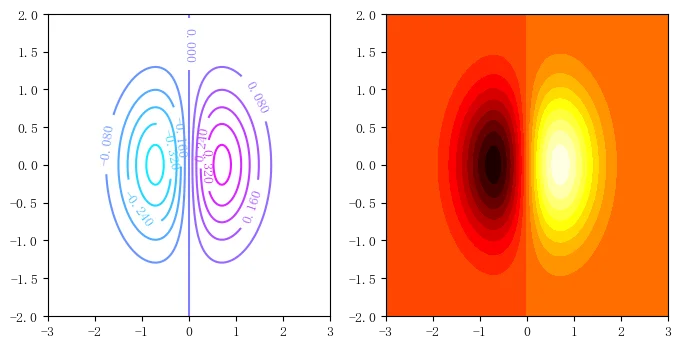• 为了更清楚地区分X轴和Y轴，这里让他们的取证范围和等分次数均不相同。这样得到的数组Z的形状为(200, 300), 他的第0轴对应Y轴， 第1轴对应X轴。
• 调用contour()绘制数组z的等值线图，第二个参数为10，表示将整个函数的取值方位分为10个区间，即显示的等值线图中将有9条等值线图。可以使用extent参数指定等值线图的X轴和Y轴的数据范围。
• 调用contourf()，绘制将取值范围分为20份、带填充效果的等值线图。这里演示了另一种设置X,Y轴取值范围的方法，他的前两个参数分别是计算数组z时所使用的X轴Y轴上的取样点，这两个数组必须是一维的。

### 4.2 二维数据的平面色彩显示

``````import numpy as np
import matplotlib.pyplot as plt
data = np.clip(np.random.randn(5, 5), -1, 1)
plt.subplot(221)
plt.imshow(data)
plt.subplot(222)
plt.imshow(data, cmap=plt.cm.cool)
plt.subplot(223)
plt.imshow(data, cmap=plt.cm.hot)
plt.colorbar() # 显示显色对应的数据的指示条
plt.subplot(224)
plt.imshow(data, cmap=plt.cm.winter)
plt.colorbar(im, cmap=plt.cm.winter, ticks=[-1, 0, 1])
plt.show()  ``````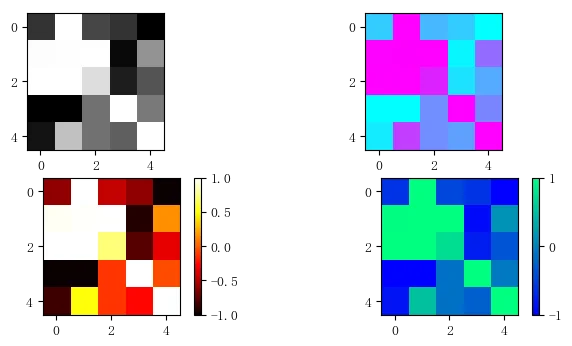## 5 3D绘图

mpl_toolkits.mplot3工具包提供了一些基本的3D绘图功能，能支持的图表类型包括散点图(scatter)、曲面图(surf)、线图(line)和网格图(mesh)。虽然mplot3d不是一个最好的绘图库，但他是伴随matplotlib产生的，一次我们对其接口已经很熟悉了。

``````import numpy as np
import matplotlib.pyplot as plt
import mpl_toolkits.mplot3d

x, y = np.mgrid[-2:2:50j, -2:2:50j]
z = x*np.exp(-x**2-y**2)
ax = plt.subplot(111, projection='3d')
ax.plot_surface(x, y, z, rstride=2, cstride=1, cmap=plt.cm.coolwarm, alpha=0.8)
ax.set_xlabel('x')
ax.set_ylabel('y')
ax.set_zlabel('z')
plt.show()  ``````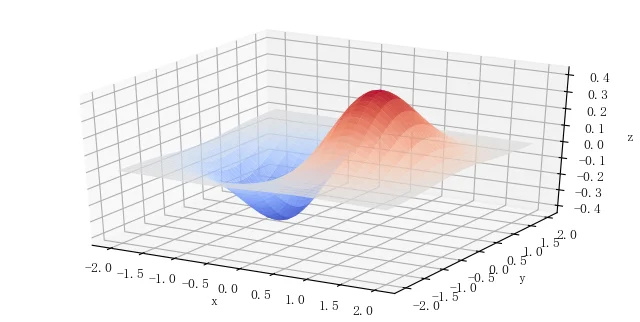目录